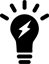6.2.10

### Confirming that the FortiGate-7000F is synchronized

In addition to viewing configuration synchronization status from the Security Fabric dashboard widget, you can use the following command to confirm that the configurations of the FIMs and FPMs are synchronized:

diagnose sys confsync status

The command shows the HA and configuration synchronization (confsync) status of the FIMs and FPMs. For each FIM and FPM, `in_sync=1` means the component is synchronized and can operate normally. If any component is out of sync, the command output will include `in_sync=0`. All components must be synchronized for the FortiGate-7000F to operate normally.To confirm the configuration synchronization status of an HA cluster, see Confirming that the FortiGate-7000F HA cluster is synchronized .

#### FIM confsync status

The `diagnose sys confsync status` command output begins with the confsync status for each FPM. In the following example for a FortiGate-7121F, the output begins with the confsync status if the FPM in slot 3. The two lines that begin with serial numbers and end with `in_sync=1` indicate that the FPM (serial number FPM20FTB21900186) is synchronized with the primary FIM (serial number FIM21FTB21000068) and the primary FIM is synchronized with the FPM.

```diagnose sys confsync status
...
Slot: 3  Module SN: FPM20FTB21900186
ELBC: svcgrp_id=1, chassis=1, slot_id=3
ELBC HB devs:
elbc-ctrl1: active=1, hb_count=52136
elbc-ctrl2: active=1, hb_count=52131
ELBC mgmt devs:
b-chassis: mgmtip_set=1

zone: self_idx:2, master_idx:1, ha_master_idx:255, members:3
FPM20FTB21900186, Slave, uptime=52134.23, priority=16, slot_id=1:3, idx=2, flag=0x4, in_sync=1
FIM21FTB21000063, Slave, uptime=52290.07, priority=2, slot_id=1:2, idx=0, flag=0x0, in_sync=1
b-chassis: state=3(connected), ip=169.254.2.16, last_hb_time=52536.01, hb_nr=260584
FIM21FTB21000068, Master, uptime=52293.10, priority=1, slot_id=1:1, idx=1, flag=0x0, in_sync=1
b-chassis: state=3(connected), ip=169.254.2.15, last_hb_time=52536.02, hb_nr=260611```

#### Primary FIM confsync status

The `diagnose sys confsync status` command output ends with the confsync status of the primary FIM, which shows the configuration status between the primary FIM and the other FIM and FPMs:

```Current slot: 1  Module SN: FIM21FTB21000068
ELBC: svcgrp_id=1, chassis=1, slot_id=1

zone: self_idx:1, master_idx:1, ha_master_idx:255, members:12
FIM21FTB21000068, Master, uptime=52293.10, priority=1, slot_id=1:1, idx=1, flag=0x0, in_sync=1
FIM21FTB21000063, Slave, uptime=52290.07, priority=2, slot_id=1:2, idx=0, flag=0x0, in_sync=1
b-chassis: state=3(connected), ip=169.254.2.16, last_hb_time=52539.80, hb_nr=261346
FPM20FTB21900165, Slave, uptime=52141.10, priority=17, slot_id=1:4, idx=2, flag=0x64, in_sync=1
b-chassis: state=3(connected), ip=169.254.2.4, last_hb_time=52539.82, hb_nr=260625
FPM20FTB21900168, Slave, uptime=52119.65, priority=24, slot_id=1:11, idx=3, flag=0x64, in_sync=1
b-chassis: state=3(connected), ip=169.254.2.11, last_hb_time=52539.83, hb_nr=260530
FPM20FTB21900170, Slave, uptime=52122.62, priority=18, slot_id=1:5, idx=4, flag=0x64, in_sync=1
b-chassis: state=3(connected), ip=169.254.2.5, last_hb_time=52539.70, hb_nr=260530
FPM20FTB21900179, Slave, uptime=52140.06, priority=19, slot_id=1:6, idx=5, flag=0x64, in_sync=1
b-chassis: state=3(connected), ip=169.254.2.6, last_hb_time=52539.79, hb_nr=260630
FPM20FTB21900182, Slave, uptime=52128.14, priority=25, slot_id=1:12, idx=6, flag=0x64, in_sync=1
b-chassis: state=3(connected), ip=169.254.2.12, last_hb_time=52539.72, hb_nr=260569
FPM20FTB21900186, Slave, uptime=52134.23, priority=16, slot_id=1:3, idx=7, flag=0x64, in_sync=1
b-chassis: state=3(connected), ip=169.254.2.3, last_hb_time=52539.84, hb_nr=260605
FPM20FTB21900189, Slave, uptime=52129.25, priority=22, slot_id=1:9, idx=8, flag=0x64, in_sync=1
b-chassis: state=3(connected), ip=169.254.2.9, last_hb_time=52539.81, hb_nr=260580
FPM20FTB21900201, Slave, uptime=52131.02, priority=20, slot_id=1:7, idx=9, flag=0x64, in_sync=1
b-chassis: state=3(connected), ip=169.254.2.7, last_hb_time=52539.74, hb_nr=260570
FPM20FTB21900203, Slave, uptime=52124.18, priority=21, slot_id=1:8, idx=10, flag=0x64, in_sync=1
b-chassis: state=3(connected), ip=169.254.2.8, last_hb_time=52539.74, hb_nr=260550
FPM20FTB21900211, Slave, uptime=52139.80, priority=23, slot_id=1:10, idx=11, flag=0x64, in_sync=1
b-chassis: state=3(connected), ip=169.254.2.10, last_hb_time=52539.85, hb_nr=260631```

### Confirming that the FortiGate-7000F is synchronized

In addition to viewing configuration synchronization status from the Security Fabric dashboard widget, you can use the following command to confirm that the configurations of the FIMs and FPMs are synchronized:

diagnose sys confsync status

The command shows the HA and configuration synchronization (confsync) status of the FIMs and FPMs. For each FIM and FPM, `in_sync=1` means the component is synchronized and can operate normally. If any component is out of sync, the command output will include `in_sync=0`. All components must be synchronized for the FortiGate-7000F to operate normally.To confirm the configuration synchronization status of an HA cluster, see Confirming that the FortiGate-7000F HA cluster is synchronized .

#### FIM confsync status

The `diagnose sys confsync status` command output begins with the confsync status for each FPM. In the following example for a FortiGate-7121F, the output begins with the confsync status if the FPM in slot 3. The two lines that begin with serial numbers and end with `in_sync=1` indicate that the FPM (serial number FPM20FTB21900186) is synchronized with the primary FIM (serial number FIM21FTB21000068) and the primary FIM is synchronized with the FPM.

```diagnose sys confsync status
...
Slot: 3  Module SN: FPM20FTB21900186
ELBC: svcgrp_id=1, chassis=1, slot_id=3
ELBC HB devs:
elbc-ctrl1: active=1, hb_count=52136
elbc-ctrl2: active=1, hb_count=52131
ELBC mgmt devs:
b-chassis: mgmtip_set=1

zone: self_idx:2, master_idx:1, ha_master_idx:255, members:3
FPM20FTB21900186, Slave, uptime=52134.23, priority=16, slot_id=1:3, idx=2, flag=0x4, in_sync=1
FIM21FTB21000063, Slave, uptime=52290.07, priority=2, slot_id=1:2, idx=0, flag=0x0, in_sync=1
b-chassis: state=3(connected), ip=169.254.2.16, last_hb_time=52536.01, hb_nr=260584
FIM21FTB21000068, Master, uptime=52293.10, priority=1, slot_id=1:1, idx=1, flag=0x0, in_sync=1
b-chassis: state=3(connected), ip=169.254.2.15, last_hb_time=52536.02, hb_nr=260611```

#### Primary FIM confsync status

The `diagnose sys confsync status` command output ends with the confsync status of the primary FIM, which shows the configuration status between the primary FIM and the other FIM and FPMs:

```Current slot: 1  Module SN: FIM21FTB21000068
ELBC: svcgrp_id=1, chassis=1, slot_id=1

zone: self_idx:1, master_idx:1, ha_master_idx:255, members:12
FIM21FTB21000068, Master, uptime=52293.10, priority=1, slot_id=1:1, idx=1, flag=0x0, in_sync=1
FIM21FTB21000063, Slave, uptime=52290.07, priority=2, slot_id=1:2, idx=0, flag=0x0, in_sync=1
b-chassis: state=3(connected), ip=169.254.2.16, last_hb_time=52539.80, hb_nr=261346
FPM20FTB21900165, Slave, uptime=52141.10, priority=17, slot_id=1:4, idx=2, flag=0x64, in_sync=1
b-chassis: state=3(connected), ip=169.254.2.4, last_hb_time=52539.82, hb_nr=260625
FPM20FTB21900168, Slave, uptime=52119.65, priority=24, slot_id=1:11, idx=3, flag=0x64, in_sync=1
b-chassis: state=3(connected), ip=169.254.2.11, last_hb_time=52539.83, hb_nr=260530
FPM20FTB21900170, Slave, uptime=52122.62, priority=18, slot_id=1:5, idx=4, flag=0x64, in_sync=1
b-chassis: state=3(connected), ip=169.254.2.5, last_hb_time=52539.70, hb_nr=260530
FPM20FTB21900179, Slave, uptime=52140.06, priority=19, slot_id=1:6, idx=5, flag=0x64, in_sync=1
b-chassis: state=3(connected), ip=169.254.2.6, last_hb_time=52539.79, hb_nr=260630
FPM20FTB21900182, Slave, uptime=52128.14, priority=25, slot_id=1:12, idx=6, flag=0x64, in_sync=1
b-chassis: state=3(connected), ip=169.254.2.12, last_hb_time=52539.72, hb_nr=260569
FPM20FTB21900186, Slave, uptime=52134.23, priority=16, slot_id=1:3, idx=7, flag=0x64, in_sync=1
b-chassis: state=3(connected), ip=169.254.2.3, last_hb_time=52539.84, hb_nr=260605
FPM20FTB21900189, Slave, uptime=52129.25, priority=22, slot_id=1:9, idx=8, flag=0x64, in_sync=1
b-chassis: state=3(connected), ip=169.254.2.9, last_hb_time=52539.81, hb_nr=260580
FPM20FTB21900201, Slave, uptime=52131.02, priority=20, slot_id=1:7, idx=9, flag=0x64, in_sync=1
b-chassis: state=3(connected), ip=169.254.2.7, last_hb_time=52539.74, hb_nr=260570
FPM20FTB21900203, Slave, uptime=52124.18, priority=21, slot_id=1:8, idx=10, flag=0x64, in_sync=1
b-chassis: state=3(connected), ip=169.254.2.8, last_hb_time=52539.74, hb_nr=260550
FPM20FTB21900211, Slave, uptime=52139.80, priority=23, slot_id=1:10, idx=11, flag=0x64, in_sync=1
b-chassis: state=3(connected), ip=169.254.2.10, last_hb_time=52539.85, hb_nr=260631```